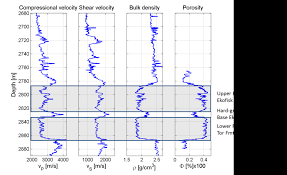## How to Calculate and Solve for Compressional or P Waves | Rock MechanicsThe image above represents compressional or P waves.

To compute for compressional or P waves, three essential parameters are needed and these parameters are Dynamic Bulk Modulus of Elasticity (kd), Dynamic Modulus of Rigidity (Gdand Elasticity of Rock Mass (p).

The formula for calculating compressional or P waves:

vp = √[kd + 4/3Gd] / p

Where:

vp = Compressional or P Waves
kd = Dynamic Bulk Modulus of Elasticity
Gd = Dynamic Modulus of Rigidity
p = Elasticity of the Rock Mass

Let’s solve an example;
Given that the dynamic bulk modulus of elasticity is 11, the dynamic modulus of rigidity is 30 and the elasticity of the rock mass is 23. Find the compressional or P waves?

This implies that;

kd = Dynamic Bulk Modulus of Elasticity = 11
Gd = Dynamic Modulus of Rigidity = 30
p = Elasticity of the Rock Mass = 23

vp = √[kd + 4/3Gd] / p
vp = √[11 + 4/3(30)] / 23
vp = √[11 + 40] / 23
vp = √ / 23
vp = 7.141 / 23
vp = 0.310

Therefore, the compressional or P waves is 0.310.